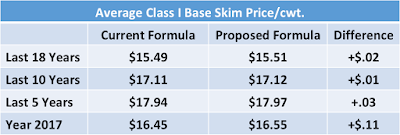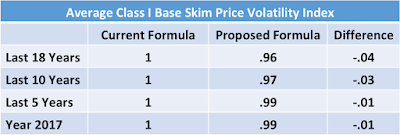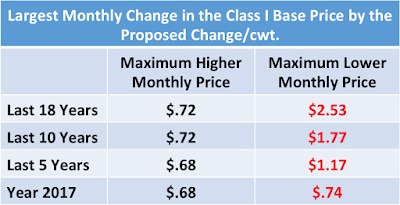## Sunday, December 3, 2017

### The Proposed Change in the Class I Price Formula-Is it Good for Producers?

The proposed change in the Class I formula for computing the price was reviewed in this blog on October 1.  Now that advanced pricing data for the full year of 2017 is available, a more complete analytical analysis is possible.   Because the Class I category is the second largest of the four Classes of Milk, any change will be impactful.  This post will review the impact of the change from the point of view of a producer.Chart I - Pie of Milk Volume by Milk Class - 2916
The current formula for determining the base Class I advanced price is the "Higher of Advanced Class III or IV Skim Milk Pricing Factors."  The proposed formula is the "Average of the Class III and Class IV Skim Milk Pricing Factors plus \$.74."  The purpose is to allow improved hedging to exactly match the formula pricing.  Because the Class III and Class IV factors can be hedged on the CME, the two can be hedged exactly.  When the formula says that the price is the "higher of", hedging can be may not be accurate because the hedging had to bet on one or the other of the Class III or Class IV price.  For those who hedge (primarily processors) this formula change will improve their predictability via hedging.

The current and proposed formulas were analyzed for four different time periods.   They are as follows:
1. The18 years from 2000 to 2017
2. The last ten years
3. The last five years
4. The current year of 2017
Average Class I base skim prices were calculated for both formulas, the current and the proposed.  Additional, the volatility of the Class I base skim price was calculated for current and proposed formulas based on traditional methods of measuring volatility.  Volatility is typically measured by comparing the standard deviation of the data for each case.   The change is then expressed as an index.

Other parameters were measured as well, such as frequency of use of Class III or Class IV in the current formulas, and trends in the frequency, impact of the proposed formula change on monthly pricing.

AVERAGE PRICE
Table I compares the Class I base price averages for the time periods listed above.  In each comparison, the average price is higher by the proposed formula than by the current formula.  The changes for just 2017 were up by \$.11/cwt., but for any longer period, the change was insignificant.  From a producer's point of view, based on Table I, there is no reason to resist the change to the proposed formula.Table I - Change in Average Class I Base Skim Price

PRICE VOLATILITY
Table II compares the impact of volatility in Class I prices when calculated by the current and proposed formulas.  In each case, the volatility is less with the proposed formulas than with the current formulas.   From a producer's point of view, based on Table II, there is no reason to resist the change to the proposed formula.Table II - Volatility of Class I Base Skim Price

HIGHER VS. LOWER MONTHLY PRICES FROM NEW FORMULA
Table III compares the number of months that the proposed formula was higher or lower than the current formula.  Over the last 18 years (216 months), in 137 months, the price was higher, and in 79 months, the price was lower over the last 18 years.  Similarly, over the last ten years, the last five years, and 2017, the number of positive months outweighs the number of lower priced months.  From a producer's point of view, based on Table III, there is no reason to resist the change to the proposed formula.Table III - Number of Months Price is Higher or Lower

FREQUENCY OF CLASS III VS. CLASS IV USAGE IN CURRENT FORMULA
Table IV shows the months and percents of using Class III and Class IV prices over the trial years.  The mix seems consistent except when viewed for a single year. If the demand for butter creates too much skimmed milk, the skimmed milk may only find a home as nonfat dry milk in the international markets.  The excess could keep Class IV prices low.  In that case, the inclusion of a low Class IV price in the Class I formula could make Class I price lower.  More on this below.Table IV - Months that Class III and IV were used in Current Class I Formula

PRICE CHANGE BY MONTH WITH PROPOSED FORMULA VS. CURRENT FORMULA
Table V shows the largest variations, both positive and negative that would occur via the change in formula.  If calculated by the new proposed formula, the largest monthly increase compared to the current formula was \$.72/cwt.  However, the largest monthly decrease compared to the current formula was \$2.53/cwt.  Because the change in the average price does not significantly change and because there are more positive months than negative months, it does make sense that the negative months have to change by more than the positive months change.Table V - Largest Monthly Changes in the Class I Base Price
To further explore the impact of the new pricing formula, Chart II shows the monthly change in Class I base skim price if the proposed formula was used over the last 18 years.  As shown in Table V and Chart II, the increases in price topped out at \$.72/cwt. and the decreases in price have lows dipping to \$2.53/cwt.  The pattern of larger price changes for negative months is consistent for all date ranges in Table V.  Importantly, note that the trend line of the change remains extremely flat, indicating that the \$.74/cwt. addition to the formula in the proposed formula is reasonable and should remain reasonable  in the future.Chart II - Change is Price with Proposed Formula vs. Current Formula
From a producer's point of view, based on Table V and Chart II, there could be a reason to resist the change to the proposed formula.  If cash flow was so tight, that a significantly lower Class I price would in a single month become a disaster, than the change in the Class I formula would create the possibility of that disaster.  In most cases, producers would be able to weather a month or more of a lower price if it all evens out over the long term.

SUMMARY
In summary, there appears to be very little reason for a producer to resist the proposed change in the Class I formula.   However, to does nothing to benefit producers who do not hedge and very few producers to hedge.  A few items, such as long-term depressed prices for Class IV milk could make the proposed formula less attractive.  However, history does not currently show any long-term trend of low Class IV prices.